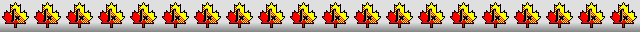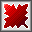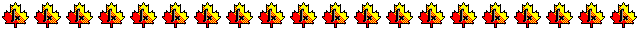Maple worksheets on the derivation of order 5 Runge-Kutta schemesDerivation of 6 stage, order 5 Runge-Kutta schemes - RKcoeff5a.mws

Derivation of 6 stage, order 5 Runge-Kutta schemes - RKcoeff5b.mws

Derivation of 6 stage, combined order 4 and 5 Runge-Kutta schemes - RKcoeff5c.mws

Derivation of 6 stage, order 5 Runge-Kutta schemes, each with a 7 stage, order 4 FSAL embedded scheme - RKcoeff5d.mws

• A stage-order 3 scheme of Prince and Dormand together with schemes that satisfy the same principal error conditions.
See: "A family of embedded Runge-Kutta formulae", by J. R. Dormand and P. J. Prince,
Journal of Computational and Applied Mathematics, Vol 6, No. 1, 1980, pages 19 to 26.
There are also two stage-order 3 schemes that do not satisfy any principal error conditions.

• Coefficients for the schemes and some properties
RKcoeff5d_1.pdf     RKcoeff5d_2.pdf     RKcoeff5d_3.pdf     RKcoeff5d_4.pdf
RKcoeff5d_5.pdf
RKcoeff5d_6.pdf     RKcoeff5d_7.pdf     RKcoeff5d_8.pdf

Derivation of 6 stage, combined order 4 and 5 Runge-Kutta schemes - RKcoeff5e.mws

• A stage-order 2 scheme of Papakostas and Papageorgiou together with other stage-order 2 schemes.
See: "A Family of Fifth-order Runge-Kutta Pairs", by S.N. Papakostas and G. PapaGeorgiou,
Mathematics of Computation,Volume 65, Number 215, July 1996, Pages 1165-1181.

• Coefficients for the schemes and some properties
RKcoeff5e_1.pdf     RKcoeff5e_2.pdf     RKcoeff5e_3.pdf     RKcoeff5e_4.pdf     RKcoeff5e_5.pdf

Derivation of 6 stage, order 5 Runge-Kutta schemes, each with a 7 stage, order 4 FSAL embedded scheme - RKcoeff5f.mws

• A stage-order 2 scheme of Papakostas and Papageorgiou together with other stage-order 2 schemes.
See: "A Family of Fifth-order Runge-Kutta Pairs", by S.N. Papakostas and G. PapaGeorgiou,
Mathematics of Computation,Volume 65, Number 215, July 1996, Pages 1165-1181.

• Coefficients for the schemes and some properties
RKcoeff5f_1.pdf      RKcoeff5f_2.pdf      RKcoeff5f_3.pdf      RKcoeff5f_4.pdf      RKcoeff5f_5.pdf

Derivation of 6 stage, order 5 Runge-Kutta schemes, each with a 7 stage, order 4 FSAL embedded scheme - RKcoeff5g.mws     RKcoeff5h.mws

Derivation of 6 stage, order 5 Runge-Kutta schemes, each with a 7 stage, order 4 FSAL embedded scheme - RKcoeff5k.mws

Derivation of 6 stage, order 5 Runge-Kutta schemes, each with a 7 stage, order 4 FSAL embedded scheme - RKcoeff5m.mws     RKcoeff5n.mws

Derivation of 7 stage, combined order 4 and 5 Runge-Kutta schemes - RKcoeff5p.mws

Derivation of 7 stage, combined order 4 and 5 Runge-Kutta schemes - RKcoeff5q.mws

Procedures related to the construction of Runge-Kutta schemes - butcher.zip

Procedures for root-finding and determining minimum points  - roots.zip

Procedures for numerical integration  - intg.zip

Top of page

Main index